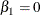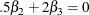### TEST Statement

TEST <"string"> equation <,equation2…>< / options> ;

The TEST statement performs Wald, Lagrange multiplier and likelihood ratio tests of linear hypotheses about the regression parameters in the preceding MODEL statement. Each equation specifies a linear hypothesis to be tested. All hypotheses in one TEST statement are tested jointly. Variable names in the equations must correspond to regressors in the preceding MODEL statement, and each name represents the coefficient of the corresponding regressor. The keyword INTERCEPT refers to the coefficient of the intercept.

The following options can be specified on the TEST statement after the slash (/):

ALL

specifies Wald, Lagrange multiplier and likelihood ratio tests.

WALD

specifies the WALD test.

LM

specifies the Lagrange multiplier test.

LR

specifies the likelihood ratio test.

The Wald test is performed by default.

The following statements illustrate the use of the TEST statement:

   proc panel;
id csid tsid;
model y = x1 x2 x3;
test x1 = 0, x2 * .5 + 2 * x3 = 0;
test_int: test intercept = 0, x3 = 0;
run;


The first test investigates the joint hypothesis thatandCurrently, only linear equality restrictions and tests are permitted in PROC PANEL. Tests and restriction expressions can be composed only of algebraic operations that involve the addition symbol (+), subtraction symbol (–), and multiplication symbol (*).

The TEST statement accepts labels that are produced in the printed output. The TEST statement can be labeled in two ways. A TEST statement can be preceded by a label followed by a colon. Alternatively, the keyword TEST can be followed by a quoted string. If both are presented, PROC PANEL uses the quoted string. In the event no label is present, PROC PANEL automatically labels the tests. If both a TEST and a RESTRICT statement are specified, the test is run with restrictions applied.

Note that for the DaSilva method, only the WALD test is available.